# TimeMoney WorldTime, Money and Growth

INVESTMENTSCOMPOUNDINGHOMESAVING

# Loan Amortisation

### The Effect of Different Rates of Interest

Here you can see how a loan of 1,000.00 (any currency) is repaid over a period of 5 years at different yearly interest rates, if the yearly interest is added to the capital at the end of each year, as it would be in a bank loan or mortgage.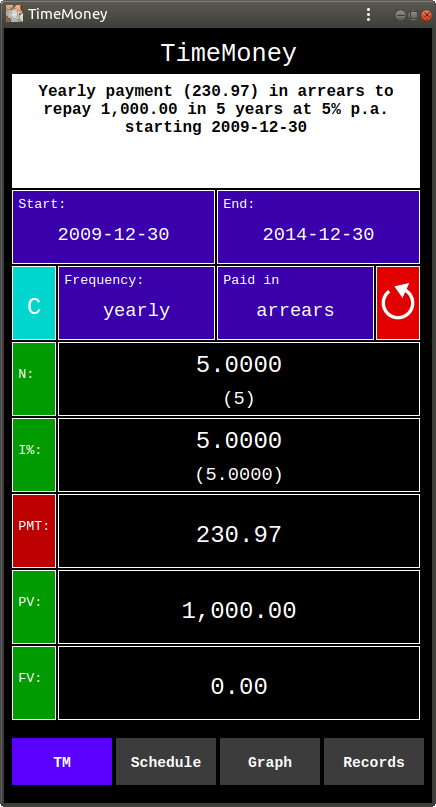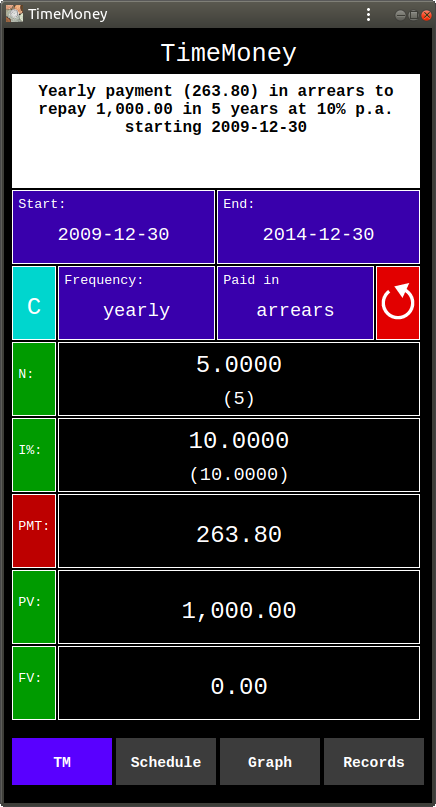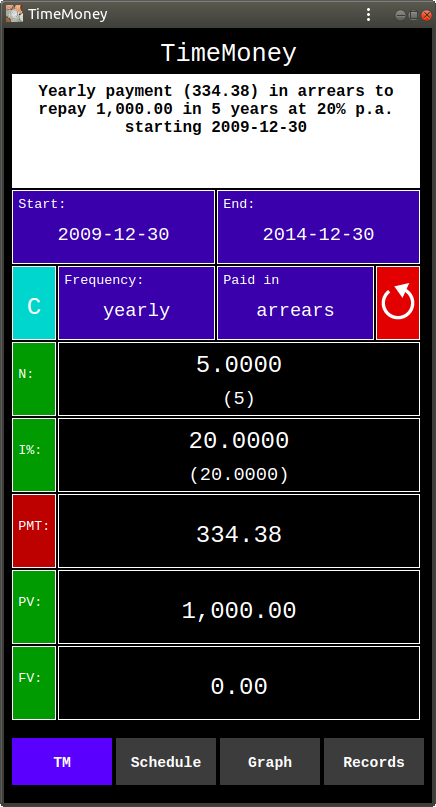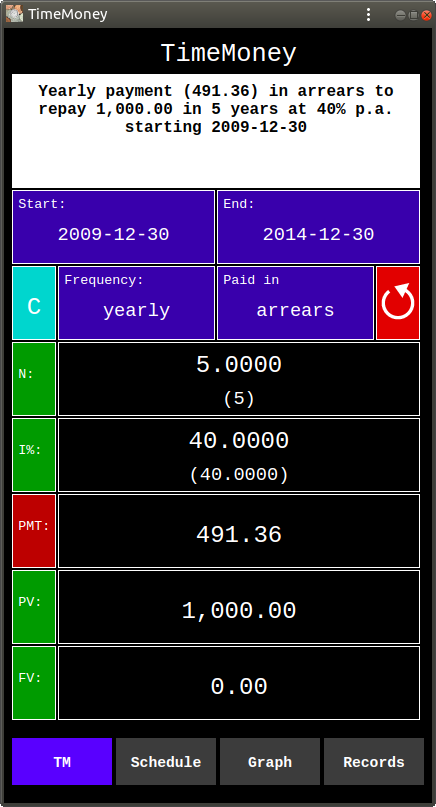Above are the computations of the Payment (PMT), required to repay a loan of 1,000.00 (PV) by 30 December 2014, which was taken on 30 December 2009, for 5 years, at different annual (yearly) rates of growth (i%): 5%, 10%, 20%, 40%.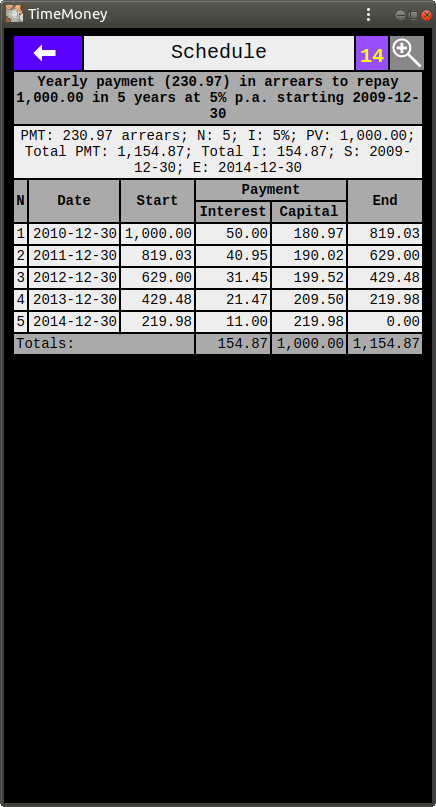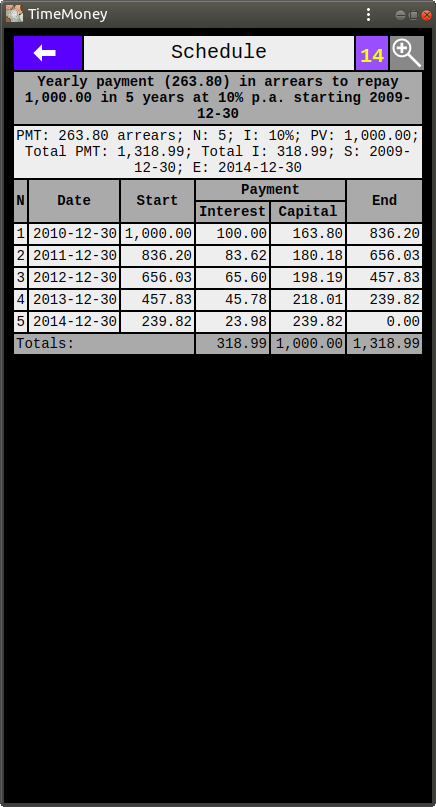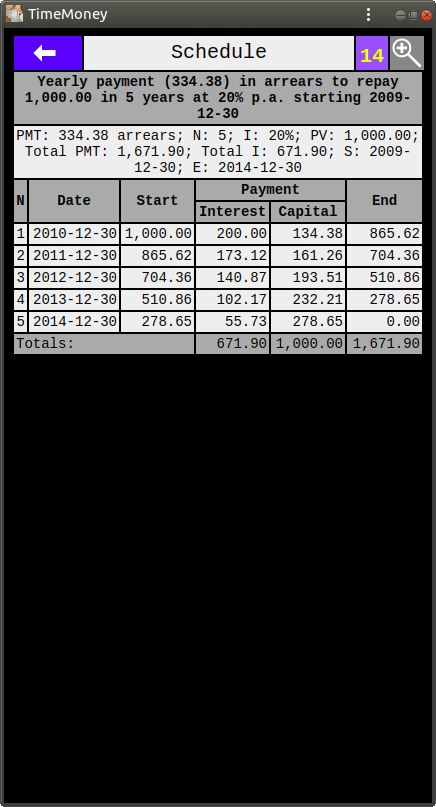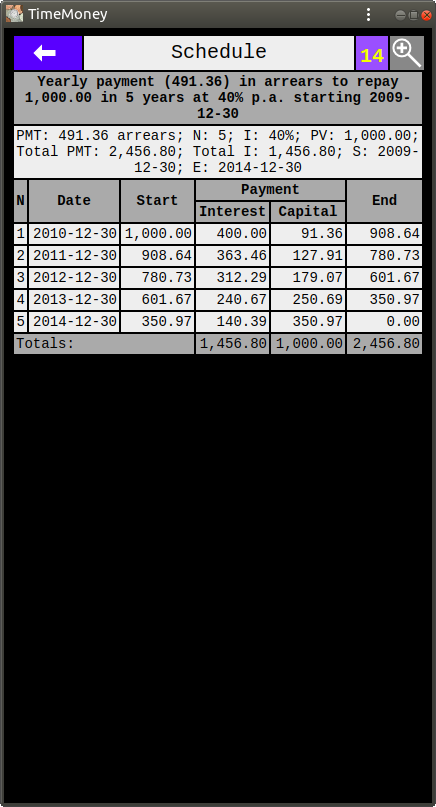Above are the loan amortisation schedules of the above computations.

The schedules show the reduction of the capital owed within each of the 5 years (compounding periods).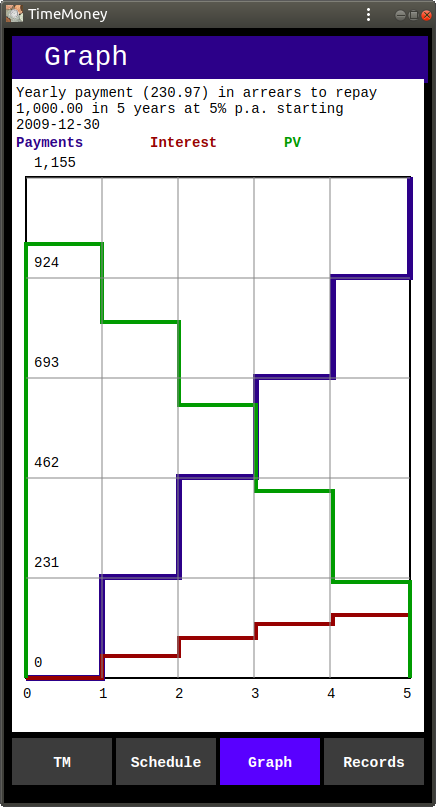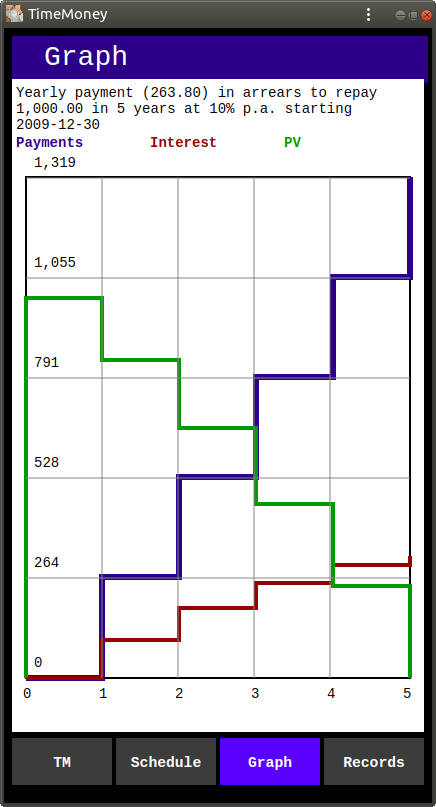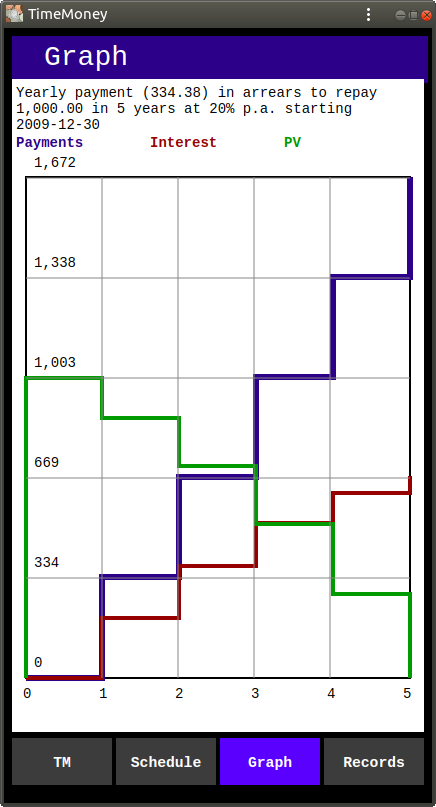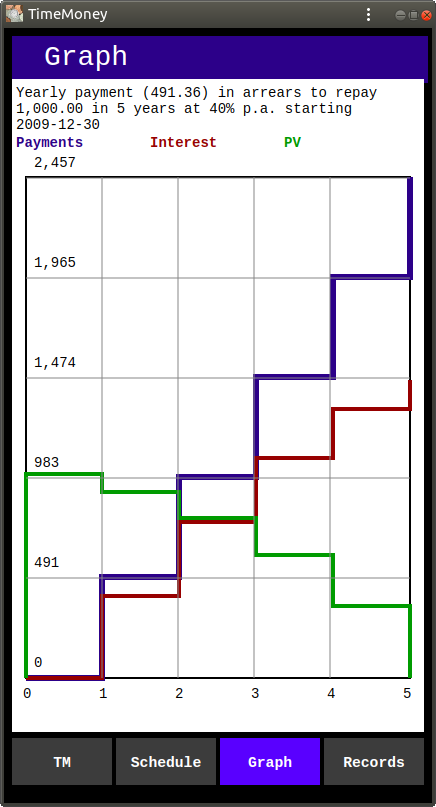Above are the graphs of the above computations.

The graphs show the reduction of the capital within each of the 5 years (compounding periods).

The green line in the graphs is the Capital (PV). The red line is the total Interest paid at the end of each period. The blue line is the total paid.

Looking at the graphs side by side, one can see that with the increase of the growth rate the part of the payment due to interest (under the red line) becomes progressively greater than the part of the payment due to capital (between the red line and the blue line).

Thus at 5% interest rate the total amount of interest paid is 15.49% of the amount of the loan, while at 40% interest the total amount of interest paid is 145.68% of the amount of the loan. That is, total interest paid is nearly 1.5 times greater than the amount of the loan.

The formula for Loan (Mortgage) Payment (PMT) is:

PMT = PV * r / (1 - (1 + r) -n)

Where:

PV is Present Value,
r is the yearly interest, as a fraction ( i / 100)
n is number of years.

The above computations, schedules, and graphs were performed using TimeMoney by Dalasoft Ltd.

TimeMoney app is a universal Progressive Web Application (PWA) which works on any device (phone, tablet, desktop) on any operating system from your browser, as long as it supports modern JavaScript. And, if you install it on your device, you will be able to start it by clicking its badge on your Home Screen, like any other app on your device.
TimeMoney app is FREE. It has no ads and does not collect any data.
To try it out, use this link: TimeMoney .